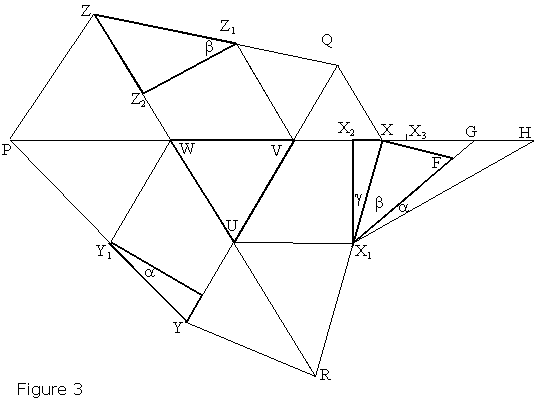## Morley's Theorem, a Proof

### Brian Stonebridge (University of Bristol, UK) Bill Millar

In Figure 1, the near trisectors of the internal angles at the vertices A, B, and C of a triangle meet in X, Y, and Z. Morley's theorem states that the triangle XYZ is equilateral. We give here a direct Euclidean proof.Let the far trisectors meet in P, Q, and R. The following proof that PX, QY, and RZ are concurrent is purely Euclidean; a stronger result can be proved using projective geometry. Figure 1 shows the angle values α, β, γ, implying α + β + γ = π/3, and ∠QXR = π - β - γ. Since Y is the incentre of ΔAQC, it follows that ∠ZQY = ∠XQY = β + π/6. The corresponding angles at R and P are γ + π/6, α + π/6.

Assume PX, QY and RZ meet in pairs at U, V, and W. By transitivity, either U, V, W all coincide or are all distinct. We show that the assumption that the three points are distinct leads to a contradiction. Indeed, this might happen in two ways, as illustrated in Figures 2 and 3 (below.) We focus on the diagram of Figure 2, the other one being entirely analogous. Sum the angles of quadrilateral QURX, using the above results, to find ∠WUV = π/3. Hence ΔWUV is equilateral, with sides 2d, say. Thus we assume that

 (1) d ≠ 0.

Choose X1 on QX such that UX1 is parallel to PX. Then the angles ZUQ, QUX1, X1UV all are π/3. Now choose X2 and X3 on PX such that ∠PX2X1 = π/2, and X1X3 is parallel to UV. These constructions imply ∠X2X1X = β (because in ΔQWX, ∠WQX = β + π/6 and ∠QWX = π/3 hence ∠QXW = π/2 - β) and X2X3 = d - from the choice of X3.Denote X2X by x, then VX = VX3 - d + x. But VX3 = UX1 = UZ, from the congruence of triangles ZUQ and X1UQ. (These triangles are congruent since they have a common side UQ, the angles at Q are β + π/6, and the angles at U are π/3.) Hence

 (2) VX = UZ - d + x.

Similarly, defining y and z in the same way as for x,

 (3) WY = VX - d + y, (4) UZ = WY - d + z.

 (5) x + y + z = 3d.

Now extend the line PX to H, such that ∠X2X1H = π/3, and hence X2H = 3d. Indeed, in an equilateral triangle the ratio of the altitude to a half-side equals 3. In ΔUVW, this means that X1X2 = d × 3. In ΔX1X2H (which is a half of an equilateral triangle), we have X2H = X1X2 × 3. Multiplying through gives

 X2H = X1X2 × √3 = (d × √3) × √3 = 3d.

Choose G on X2H such that ∠XX1G = γ. Then ∠GX1H = α. Finally, choose F on X1G such that XF is normal to XX1. Then ΔXX1F and ΔY2Y1Y are similar. Since XX1 > X2X1 = Y2Y1, then XF > y. Because ∠XFG = π/2 + γ, it is the greatest angle in ΔXFG. Hence, by Euclid (I.19), XG is the longest side, so y < XG. Similarly, z < GH. Including x = X2X, it follows that x + y + z < 3d. This contradicts (5) and so the assumption d ≠ 0 was false. That is, PX, QY, and RZ are concurrent, with mutual angles of π/3.

Now complete the proof of Morley's Theorem. As was observed earlier, in Figure 2, UZ = UX1, so that ΔZUX1 is isosceles. Hence YQ, being the bisector at vertex U, is normal to the base ZX1. But X1 and X now the same point, so ZX is normal to YQ. Similarly, XY, YZ are normal to RZ, PX, implying that the ΔXYZ is equilateral.### Morley's Miracle

#### Invalid proofs